# Derivation of the Perspective Matrix, Part 1

Preface

I have been wanting for some time to discuss in detail the Perspective Matrix. Not only do I find it an interesting topic but I have found numerous times during my career that it has helped to understand the Perspective Matrix and the math behind how it came into being. I'm not going to assume too much knowledge of the subject in this article so I'll start with the basics. Skip a bit if it's of no interest to you but I do hope that others will find this particular granularity of breakdown useful. I know it's been years since I've formally looked into the math behind this and I certainly befitted greatly from trying to explain how the perspective matrix works to others.

While this piece is intended to be read all together I decided to break it up into several easy to digest parts more suitable to appearing on a development log. It's entirely possible I've made mistakes or omissions and I invite readers to point them out. I, like everybody, is learning all the time.

Part 1

Since the gaming world went 3D the perspective matrix has come into it's element. Its the math which takes our 3D game world and displays it on our 2D televisions, monitors and screens. There are two common types of 3D projection, orthographic and perspective. Orthographic projection has it's place but for the most part it's perspective projection that does the heavy lifting in game titles.

Artists have been aware of the phenomena of perspective for thousands of years. In particular with regards to the apparent relative size differences of objects depending on their distance from the viewer. A good artist depicts perspective intuitively and artistically.

Over time mathematicians and natural scientists developed theories about perspective. The mathematics we use today seem to do a very good job of simulating perspective as we see it in the real world. Using linear algebra and the associated matrix math we can handily simulate a camera in our virtual 3D worlds.

While we are in the moment less interested in orthographic projection it does serve to understand a little about it and it's differences to perspective projection. An orthographic projection will map a 3D point on to a 2D surface by modeling how the light travels from a 3D point to the 2D surface. The ray of light will intersect the projection surface orthogonally to that surface, in other words with a right angle – a perfect 90 degrees to the 2D surface. You could think of this as a lot like shadows projected on to a wall (an ray of anti-light if you like); as if you were playing with shadow puppets. The 2D surface will need to be at least as big as the 3D object in order to receive the projected rays at 90 degrees (in the natural world – in mathematics we are able to scale things).

A camera, or an eye for that matter, is different, and a perspective projection will more accurately apply in this case. To understand why, one has to imagine what the light might be doing in order to reach the lens of the eye or camera. The viewing eye is quite small, so light that is viewed will be the light rays that will travel from the 3D point to the point where the eye is located. There is no condition on the light having to strike the 2D viewing surface orthogonally. In effect light will arrive at different angles on the 2D plane depending on its source distance and orientation. This is where the visual effect of perspective comes into existence. This is obviously simplified as an eye and a camera have a lens which changes the way the light travels, but we can ignore that for now.

In your typical game, world co-ordinates are 3D co-ordinates relative to an arbitrary world origin. Camera or eye co-ordinates are 3D co-ordinates which are relative to an origin that is specified as being the position of the eye or camera.

If we setup a little thought experiment and layout a diagram on paper or computer we can start to see where the mathematics will come from. Let us assume the geometry we're dealing with (points, lines and so on) has already been transformed from world co-ordinates to eye co-ordinates and that the projection plane is the same as the front clipping plane. It's not really relevant to this discussion so we'll ignore how that happens for now.

If you reference the figure below you will be able to see how we imagine this model of our camera to be. A Right Handed Co-ordinate system is in place. We are using a viewing frustum aligned with the Z axis (the Z axis is relative to the eye so we subscript it with the lowercase e), with a near/front clipping plane at Ze = D, and far clipping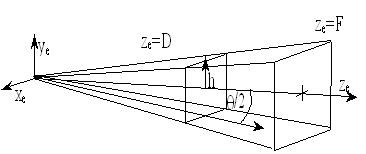plane at Ze = F, and the view angle we have represented by the half height of the frustum h. Note that the half height can also be represented by the viewing angle divided by 2. In this diagram we label the viewing angle with the lowercase Greek letter theta.

Let us start by working through the transformation of a point in 3D space to a point in 2D space. For simplicity sake the 2D space we're talking about is our near clipping plane. If you like, what we project on here gets displayed onto our screen as a 2D image.

As stated earlier we have agreed our 2D plane on which we are projecting is located at the near clip plane. If we want to be able to position points on this plane we need to give it a co-ordinate system of it's own. By convention this is known as screen space. Screen space being 2D will only have 2D co-ordinates, for simplicity we make the origin the center of the screen. In the figure above we can imagine it being the point at which the eye Z axis intersects our viewing plane.

Using the mathematics of projection we can establish a projected 3D points position on the 2D plane by using the formula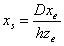To understand this equation lets look at a more simple equation. The equation is Xss = Xe * D / Ze. It looks very much like the original formula above. What we see here is simply an application of the principle of similar triangles. If the angles of one triangle are equal to the angles of another triangle, then the triangles are said to be equiangular. These equiangular triangles have the same shape but may have different sizes. So equiangular triangles are also known as similar triangles. This principle is relevant here, if you look at the figure. This principle states that the ratio of the length of the sides of two triangle remains the same for similar triangles.

This principle then allows us to see where Xss = Xe * D / Ze comes from. The two triangles we propose are similar are the triangle formed by the eye, a point on Xe and a point on Ze. The second triangle is the one formed by the eye, a point on Xs and the point D along the Ze axis. We can see they are similar triangles so thus the length ratio between the sides from the eye to D and Eye to Ze will be the same ratio between eye to Xe and screen origin to Xs. Knowing this ratio we simply multiply the ratio by the length of the side we do know about on the other triangle, yielding the length of the side we're interested in which happens to be Xss.

So now we understand where we get the first part of the projection, the part for the X axis at least. The same principle works exactly the same along the Y axis.

That part was straightforwards enough, but we know that we're still missing the h term from our simplified formula. Now lets look at where the half height of the screen comes in to the equation. The half height of the screen is another way of expressing the field of view of the camera. The field of view of the camera we signify in this example as the angle theta. It is easy to see that if we increase the value of h the screen area we have for projecting on to is much larger. Dividing Xe * D / Ze by h will yield Xs as a value in screen space.

Using basic trigonometry we can see that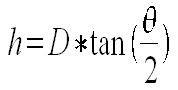So substituting this back into our equation we get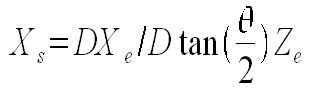which becomes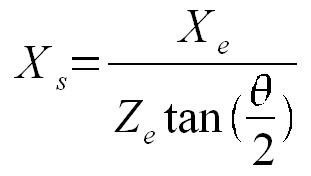and this for our Y axis component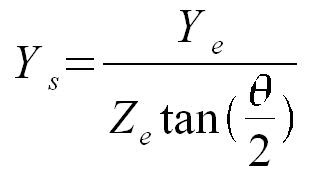So here have arrived at two simple equations for projection of a point on to the 2D plane we've decided is our screen. We can use our equations from this position in our journey to do more, which I'll cover in part 2.